# What Is an Inverting Op-Amp?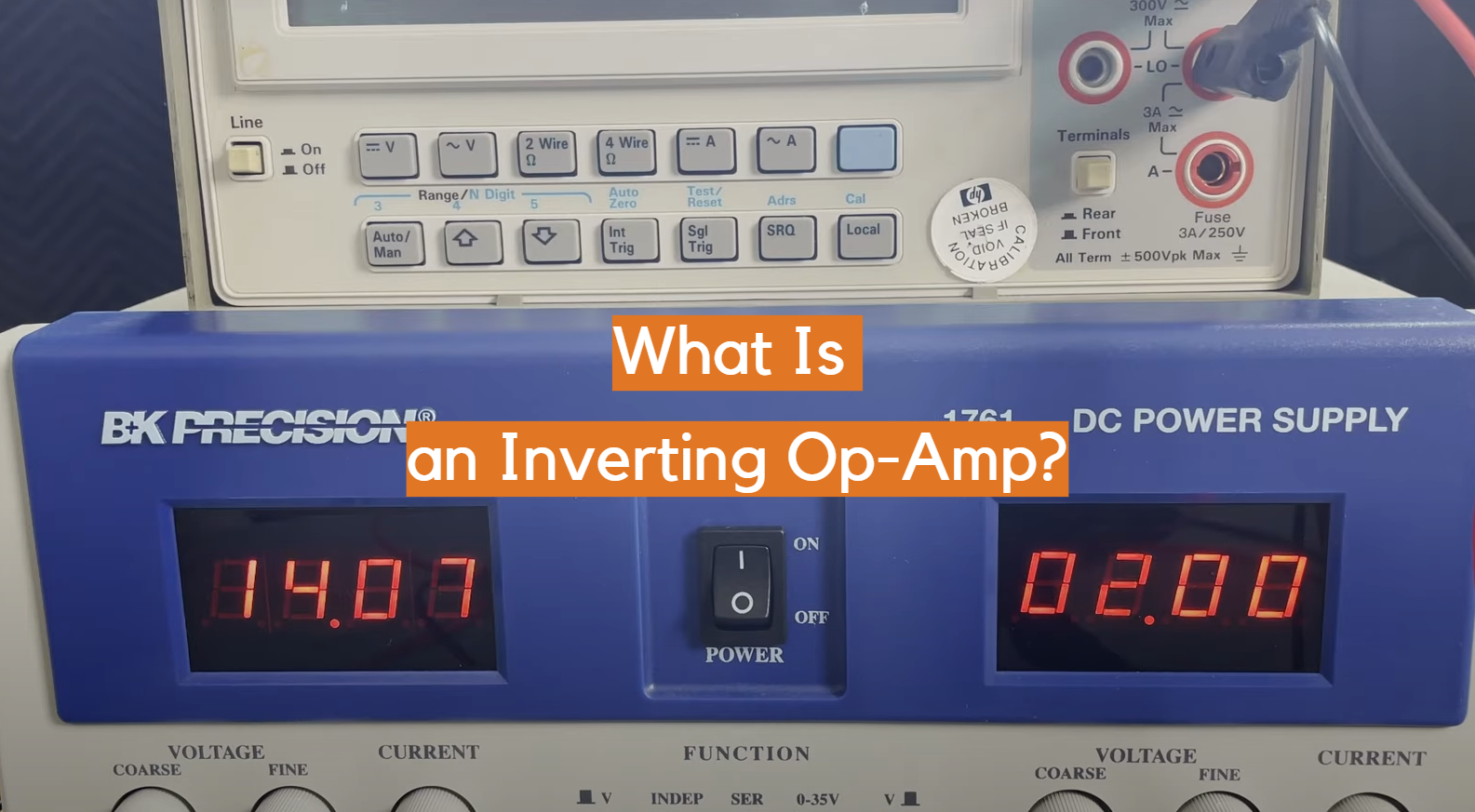An op-amp is an electronic component that has a variety of uses in circuitry. There are two main types of op-amps, inverting and noninverting. In this article, we will be focusing on the inverting type. Inverting op-amps are used to change the polarity of a signal, while noninverting op-amps amplify signals without changing their polarity. If you’re new to electronics or just want to learn more about inverting op-amps, read on!

## Definition of an Inverting Op-Amp

An operational amplifier (or “op-amp”) is a type of circuit that amplifies electrical signals. It is composed of transistors, resistors and capacitors and can be used to perform various tasks such as voltage amplification, filtering, signal conditioning, signal summing and more.

Op-amps are widely used in many industries because they are extremely versatile and robust. They are typically found in audio equipment, industrial automation systems, medical applications and test instruments.

The basic design of an op-amp consists of two inputs (inverting and non- inverting) which are connected to the same reference point known as a virtual ground. The output from the op-amp will depend on how much current flows through the circuit.

An inverting op-amp is an operational amplifier circuit that has a negative feedback connection between its output and input terminals.

It is designed to produce an output signal which is 180° out of phase with the input signal, resulting in an amplified inverted version of the input voltage when both are connected together.

The inverting op-amp can be seen as having two main components; the differential amplifier stage and the feedback loop. The differential amplifier stage amplifies the difference between two input voltages, while the feedback loop controls how much of this amplified signal will return back to the inputs in order to create a gain or attenuation depending on whether it’s positive or negative. This creates a closed loop system that is highly stable compared to open-loop operation.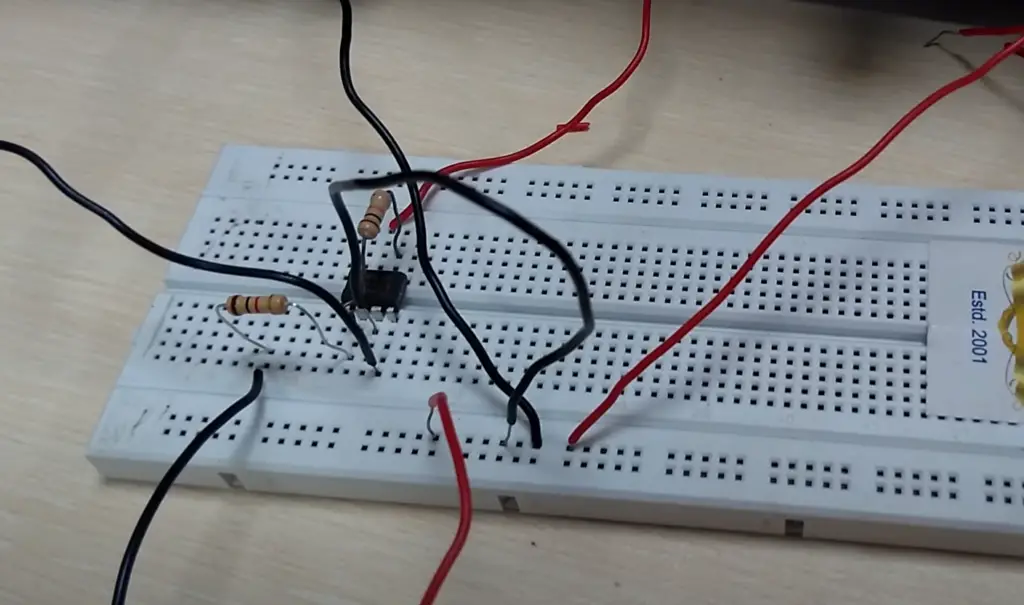The gain of an inverting op-amp can be easily calculated by multiplying the ratio of two resistors in the feedback loop.

Since its introduction, the inverting op-amp has become a popular choice for many applications such as speaker systems, filters, signal conditioning and many more. It is also used in some of the most advanced medical imaging technologies available today, due to its ability to accurately amplify signals while providing excellent linearity and low noise performance. , , , 

## How do Inverting Op Amps Work?

An inverting op-amp is a type of operational amplifier (op-amp) circuit that works by converting direct current (DC) electrical signals into an amplified output signal.

Inverting op amps are typically used in analog electronic circuits and allow for amplification or attenuation of the input signal, depending on how they are configured. The most common configuration is to have the input voltage applied to one terminal, known as the non-inverting terminal, while the output voltage is taken from another terminal, known as the inverting terminal.

When this type of circuit is wired correctly, it can be used for many different applications including signal conditioning, controlling gain and frequency response, adding distortion effects, filtering out unwanted noise and more.

One thing about the inverting amps, is that no current flows into the inputs of the op amp. This is because the input signal is applied to the inverting terminal and not to the non-inverting terminal.

The inverting op amp ensures that the voltage differential between its two inputs is kept at zero, with its output striving to reach this goal. Any current entering the input terminal provides a negative feedback that brings the output voltage down towards zero and stops any further current from entering. , , ,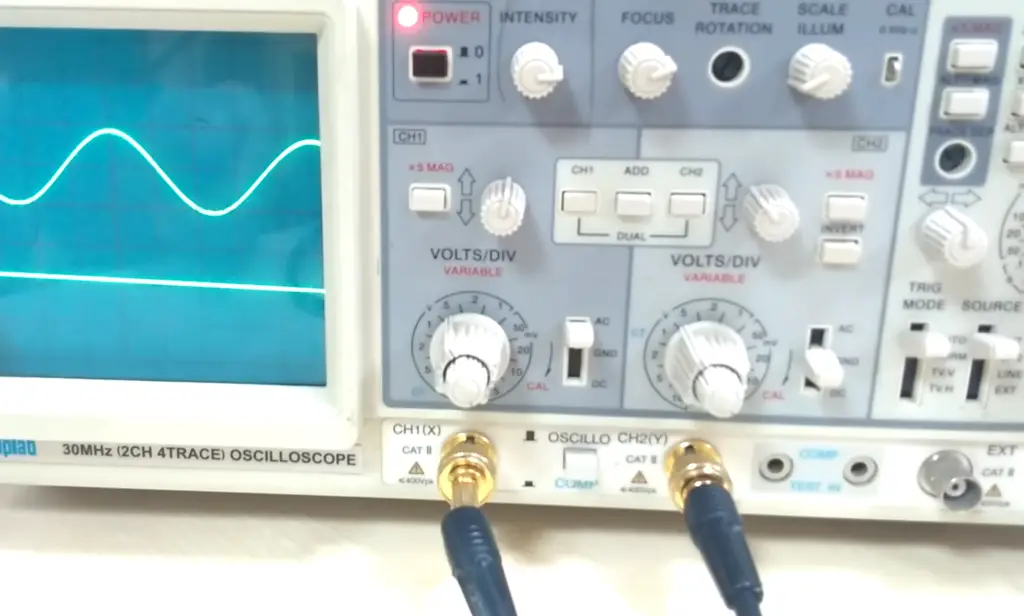## Voltage Gain of An Inverting Amplifier

Voltage gain is a measure of the amplification of an electronic circuit.

It is defined as the ratio of the output voltage to the input voltage and is usually expressed in decibels (dB). Voltage gain can be used to determine how much an amplifier or other device increases the magnitude of a signal, such as sound or electrical current.

A higher voltage gain means that more power will be delivered to the load than was supplied at the input, resulting in greater overall efficiency. Voltage gain also plays a role in determining how signals are transmitted across different media and allows users to adjust their systems for optimal performance. For example, some audio amplifiers allow users to adjust their voltage gain so that certain sounds are amplified louder than others for better clarity. Knowing how to calculate voltage gain and understanding its importance is essential for anyone who works with electronics.

Now, how do you invert? Op-amps work in terms of voltage gain? The formula for calculation is (Av = Vo/Vi). Where, Vi-V1 = IiRi and V1-V0 = IfRf.

Here, Av is the voltage gain of an inverting op-amp, Vo and Vi are the output and input voltages respectively, Ii is the current through Ri, Rf is the feedback resistor and If is the current flowing through it.

From this formula it can be seen that as Ri increases in value, the gain of an inverting op amp also increases. This means that if a higher gain is needed for a particular application, then a larger feedback resistor should be used to increase the output signal. Conversely, decreasing Ri will lead to lower gains at the output. It can also be noted that increasing Rf will have the same effect on voltage gain as increasing Ri due to their inverse relationship with one another. , , , 

Now you should have a solid understanding of what an inverting op amp is and how to calculate the voltage gain. But why would you want to use one in the first place? In this section we will discuss the advantages of using inverting op amps in your circuits compared to other types of amplifiers.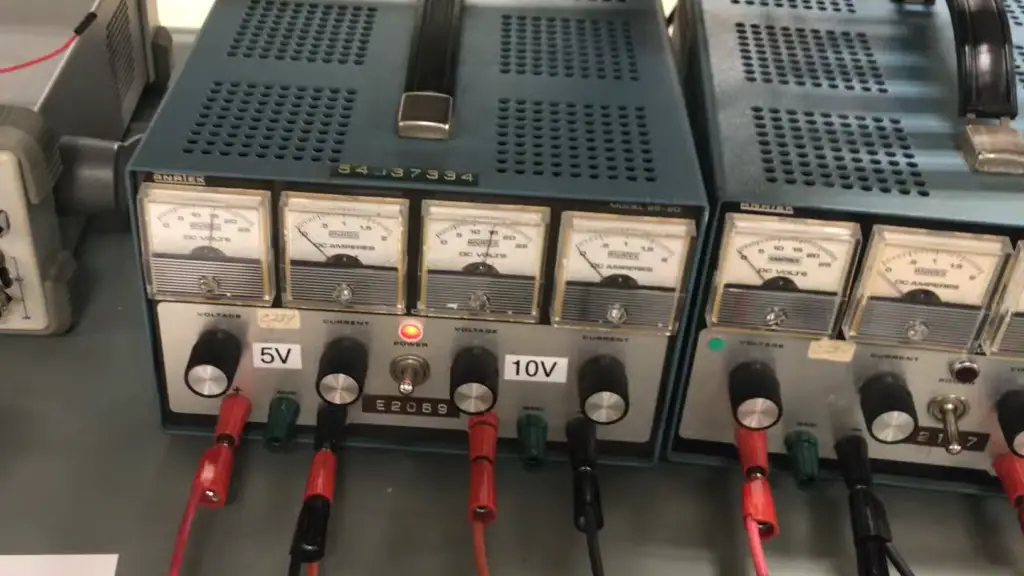### Versatile

The first advantage of using an inverting op-amp is its versatility. By connecting different resistors in the circuit, you can easily adjust the gain and other parameters as needed for various applications. This makes it easy to set up a circuit for a specific application without having to purchase multiple amplifiers with predetermined gains or bandwidths.

### Relatively affordable price

Inverting op amps are typically relatively affordable compared to other types of amplifiers. This makes them a great option for hobbyists, as it’s easy to find one at an attractive price online or from your local electronics store.

This makes them ideal for hobbyists who want to build their own circuits without breaking the bank.

### Dependable

One of the biggest advantages of inverting op amps is their dependability. This type of amplifier has been around for quite some time and there are countless examples of how reliable it can be in a variety of circuits and applications. Inverting op amps are also commonly used in instrumentation amplifiers, which require high levels of accuracy and reliability. This means that you can count on your circuits to perform consistently without worrying about them breaking down or malfunctioning due to an unreliable component.

### Small size

Inverting op amps are incredibly small and compact. This is due to their design which only requires a few components, such as the operational amplifier itself and two external resistors. This makes them suitable for use in portable or space-limited applications, where size is an important factor.

### Flexible

Inverting op amps are very flexible and can be used in a variety of circuits. They can easily provide the required gain, while also allowing you to control the frequency response of the circuit with capacitors or resistors. This makes inverting op amps ideal for designing filters and other signal processing applications. Additionally, they can also be used as oscillators, comparators, and even voltage regulators!

### Strong anti-interference capacities

Another of the major advantages of inverting op amps is their strong anti-interference capacity. When a signal passes through an inverting op amp, the output will be relatively unaffected by noise that might be present in the input signal. This makes them ideal for use in applications where signals may have to pass through a noisy environment or be subjected to other types of interference.

### High gain factor

Inverting op amps can have a high gain factor. This means that for every input voltage, the output voltage is amplified by a large amount. This makes them ideally suited for applications where you need to amplify small signals, such as amplifying audio signals from microphones. ,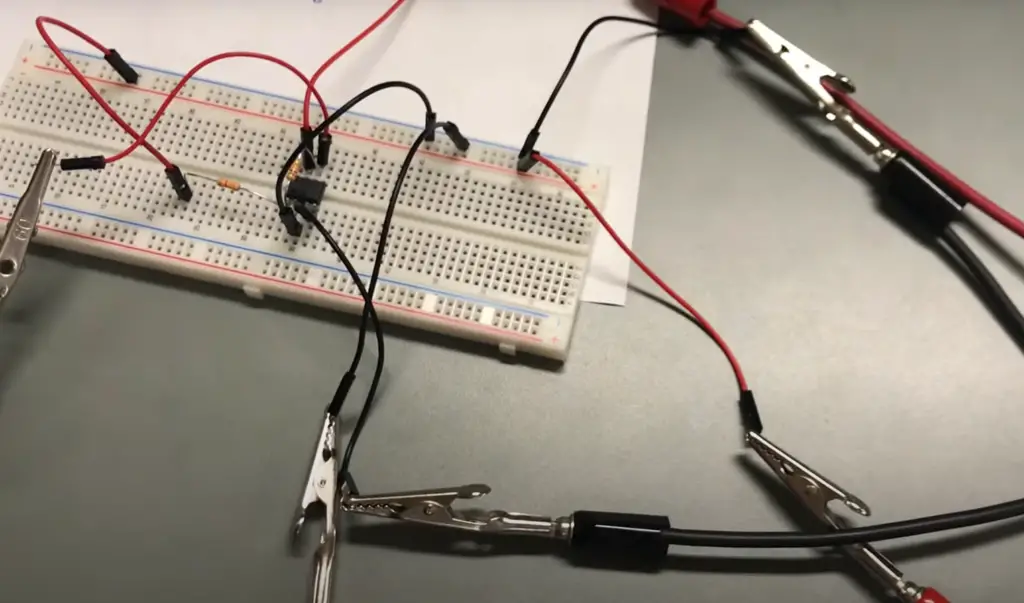Despite all the advantages of using an inverting op amp, there are also some drawbacks to consider. While some may not consider the following a dealbreaker, they should still be taken into account when making a decision.

### The signal is reversed

One of the biggest potential drawbacks of using an inverting op amp is that the signal is reversed. This means that when the input voltage increases, the output voltage decreases and vice versa. This can be problematic in some applications where a positive voltage is required from the amplifier, such as power supply circuits.

### Small input impedance

Input impedance is a measure of how much opposition an electrical circuit presents to the flow of current when voltage is applied.

It is usually represented as a complex quantity, which consists of two resistance components: resistive and reactive components. The resistive component represents the ohmic resistance to the flow of current, while the reactive component represents the effect of capacitance and inductance on the current. Input impedance is determined by the type and configuration of electronic components in a circuit, such as transistors, diodes, capacitors, and inductors.

The input impedance of an inverting op amp is relatively low. This means that it can draw more current from the signal source, which could increase distortion or reduce the overall performance of the circuit. When the input impedance of an op amp is reduced, more current must be supplied by the signal’s source.

### The input signal should not include the noise

Since the inverting op amp amplifies the input signal so much, any noise present in that signal will also be amplified. This means that if there is noise present in the input signal, it can lead to significant distortion or clipping of the output. To avoid this issue, you must make sure that your input signal does not include any noise or interference before it enters the circuit. ,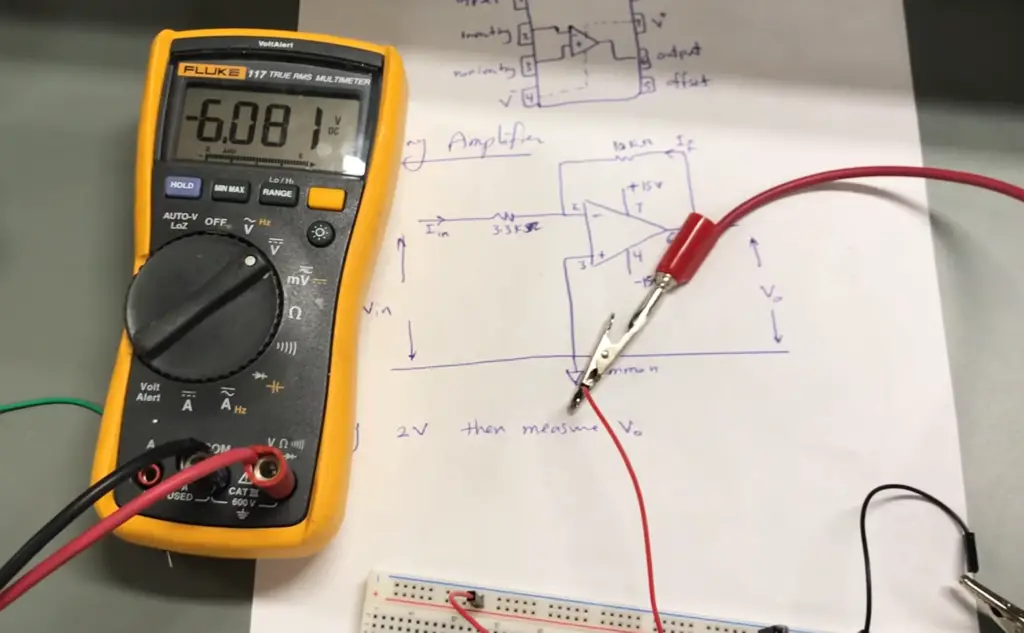## Applications of Inverting Op-Amp

Now that you know the basics of inverting op amps and their advantages and disadvantages, let’s take a look at some of the common applications for this type of amplifier.

### It can be used as a phase shifter

Inverting op amps are often used as phase shifters. A phase shift occurs when the output signal’s waveform is shifted in time with respect to the input signal’s waveform. This can be useful for applications such as filtering, equalization, and audio processing.

### It can be used instead of trans resistance amplifier

One of the most common uses for an inverting op amp is as a substitute for a trans-resistance amplifier. This type of amplifier takes an input voltage and produces a proportional resistance at its output. The advantage of using an inverting op amp instead is that it eliminates the need to use resistors, which make these circuits more cost effective.

### It can be used in applications where balancing of the signal is necessary

Inverting op amps are often used in applications where balancing of the signal is necessary. This includes audio mixing and recording, hearing aids, medical instruments, radio frequency (RF) transmitters, and high-precision thermometers. In these cases, an inverting amplifier can be used to take a single input signal and convert it into two outputs with equal magnitude but opposite phase.

### It’s used in integration applications

Inverting op amps are often used in integration applications. This is because the output voltage is proportional to the integral of the input voltage, which makes them well suited for integrating signals over time. Common integration applications include wave shaping, signal processing, and control systems.

### Signal processing

Inverting op amps are commonly used in signal processing applications, such as audio or video amplifiers. They can be used to amplify a small signal and increase its strength so that it can be heard or seen more clearly.

### It’s used in electronic devices with linear ICs

Inverting op amps are commonly used in electronic devices with linear integrated circuits (ICs). These include amplifiers, oscillators, filters, and other types of signal processing blocks. They can be used to amplify very small signals like audio signals from microphones or generate a stable output voltage even when the input voltage is highly variable. ,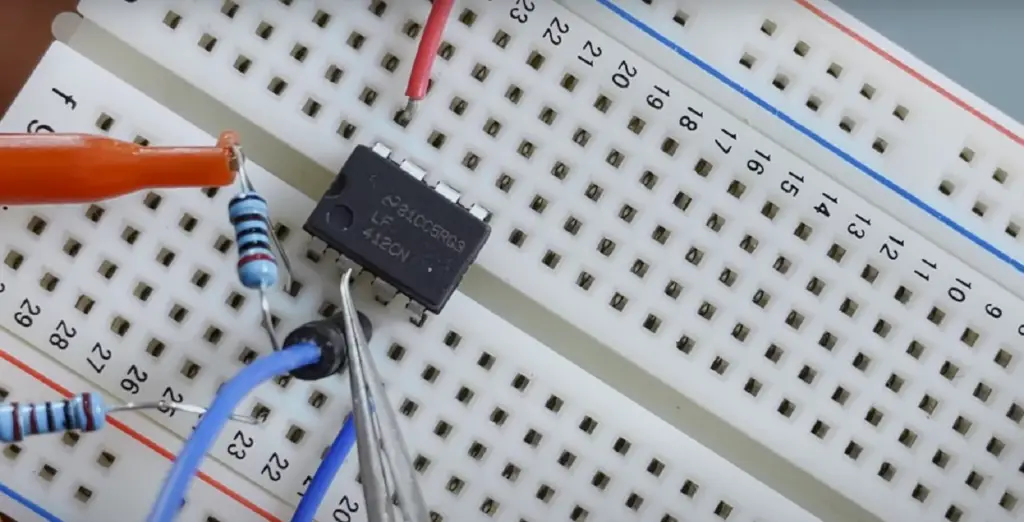## FAQ

### How do I know if my op-amp is stable?

Analyzing the loop gain of an op-amp against frequency is a simple way to determine its stability. When the loop gain equals 0 dB (gain equal 1) we’ve reached a critical point, as it must have at least one unit of gain in order to become unstable.

### Why are inverting op-amps more stable?

The inverted amplifier op amp is more popular than the non-inverting one due to its circuit set up. In this configuration, the non-inverting terminal is grounded and thus creating a virtual ground at the inverting op amp’s end. This simple setup offers tremendous advantages for all types of applications, making it an ideal choice for many users.

With inverting op-amps, the user can easily control the gain and input impedance. This is because the voltage divider of input impedances that usually forms at the non-inverting terminal does not exist in this configuration.

### Can a non-inverting op-amp gain less than 1?

No, a non-inverting op-amp is designed to only have a gain greater than 1. The gain of a non-inverting op-amp can range from 1 to infinity depending on the feedback resistor ratio and input impedance of the circuit.

This is something to keep in mind when designing a circuit as the gain of an op-amp can have a great effect on its performance. For example, if you were to use a non-inverting op-amp with no feedback resistor, then the gain would be infinite and could cause the amplifier to oscillate and become unstable.

### What is the difference between an inverting op amp and a non-inverting one?

The primary difference between an inverting op amp and a non-inverting one is their respective gains. An inverting amplifier has a gain that is equal to negative one divided by the ratio of its input impedance to output load resistance. A non-inverting amplifier has an overall gain that is equal to the ratio of its input impedance to output load resistance.

In addition, an inverting amplifier always produces an output that is 180 degrees out of phase with its input signal, while a non-inverting amplifier produces an output in phase with its input. This difference is essential for certain applications, such as active filters and phase-shifters.

### When would I use a non-inverting op-amp?

Non-inverting op-amps are commonly used when the non-inverting op-amp circuits are used where high input impedance is necessary. When the input impedance is high, this allows for greater control over the signal as it is passed through the circuit. Additionally, non-inverting op-amps allow for higher gain with lower noise levels than their inverting counterparts. Non-inverting op-amps are also used in audio circuits where an amplifier can be used to boost the volume of a sound source or when two signals need to be added together.

### What is a non-inverting op-amp?

A non-inverting op-amp is an operational amplifier (op-amp) with two inputs, one of which experiences a 180° phase shift relative to the other.

The output from a non-inverting op-amp is in phase with its input signal and has an amplified gain determined by its resistor values. Depending on the application, adjustable resistors can be used to fine tune the desired gain.

The inverting op-amp is similar to the non-inverting op amp but its output signal is out of phase with the input signal due to its 180° phase shift. As with the non-inverting amplifier, it too has adjustable resistors allowing users to make small changes in gain depending on the application they are using the amplifier for.

### Why is an inverting amplifier better?

An inverting amplifier is typically better than a non-inverting one due to its higher gain and input impedance. Inverting amplifiers are also known for their low output resistance, which allows them to drive heavy loads without causing signal degradation. Additionally, the feedback loop of an inverting amplifier helps increase stability compared to a non-inverting amplifier, as it can reduce the effects of noise on the system.

The most significant benefit of using an inverting amplifier is that it can help create negative feedback loops for precision analog circuits. This type of closed-loop topology helps ensure accuracy by reducing the effects of errors from temperature changes and other external factors. An inverting op amp can also be used in differential amplifiers, instrumentation amplifiers, and other types of active filters.

### Is inverting input positive or negative?

An inverting input is negative. This is because of the circuit configuration of an inverting op-amp. The output signal is 180° out of phase with the input signal and therefore, its polarity is reversed. The amplifier takes a positive input at one end and a negative input at the other end, which then produces a voltage output with respect to the ground that has opposite polarity as compared to the input.

## Conclusion

Inverting op-amps are an essential part of electrical engineering and provide a huge range of benefits in circuits. They can be used to amplify signals, reduce noise, and more. By understanding the basics of inverting op-amps, electrical engineers can maximize the use of these components in their projects. When setting up an inverting amplifier circuit, it is important to remember to ensure proper biasing and gain selection for best performance results.

In this article, we have discussed the basics of inverting op-amps and their uses in electrical engineering applications. We reviewed an example circuit to illustrate how an inverting op-amp can be used to amplify a signal. Finally, we covered the pros and cons of using inverting op-amps. By understanding this material, engineers can make better choices when selecting components for their projects.

Inverting op-amps are important components that offer many benefits for electrical engineers and should not be overlooked in any design project. By following the tips mentioned in this article, you will be able to make sure your inverting amplifier circuits work reliably and effectively.

With all these details in mind, you can now confidently use inverting op-amps for all your electronics projects! If you have any questions or comments, please feel free to leave them below. Thank you for reading!